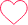# How To Solve Clocks And Calendar Questions Quickly

## Definition of Clock and Calendar

We will be discussing How to Solve Clocks and Calendar Questions Quickly to help students to increase efficiency at the time of Examination.## How to Solve Clocks and Calendar questions Quickly: Clock Type

### Question 1.

What was the day on 10th November,1581 ?

Options:

A. Thursday

B. Monday

C. Wednesday

D. Tuesday

#### Solution

10 November 1581 = (1580 years + Period from 1.1.1581 to 10.11.1581)

To calculate number of odd days till 1580, we need

Number of odd days in 1200 years = 0

Number of odd days in 300 years = 1

Number of odd days in 80 years = 20 leap years + 60 ordinary years = 2 odd days

Therefore, 1580 years had 0 + 1 + 2 = 3 odd days

Now, for calculating odd days for 1.1.1581 to 10.11.1581,

January (31 days) + February (28 days) + March (31 days) + (April 30 days) + May (31 days) + June (30 days) + July (31 days) + August (31 days) + September (30 days) + October (31 days) + November (10 days)= 314 days

Total number of odd days in 314 days = (314/7) = 6 odd days

Total Number of odd days corresponding to the given date = 3 + 6 = 9 days = 9/7 = 2 odd days

As per the table, on 10 November 1581 the day was Tuesday.

Year 2019 2020 2021 2022 2023 2024 2025 2026 2027 2028 2029
No.of Odd days 1 2 1 1 1 2 1 1 1 2 1

### Question 2.

Suppose today is Monday. Now find out what will be the day after 62 days?

Options:

A. Sunday

B. Monday

C. Wednesday

D. Tuesday

#### Solution

Each day to the week is repeated after 7 days.

Therefore, after 63 days, it will be Monday.

Which means that on 62nd day, it will be Sunday.

### Question 3.

Which year will have the same calendar as that of the year 2019?

Options

A. 2029

B. 2030

C. 2031

D. 2024

#### Solution

Count the number of odd days from the year 2019 onwards to get the sum equal to 0 odd day.

Year 2019 2020 2021 2022 2023 2024 2025 2026 2027 2028 2029
No.of Odd days 1 2 1 1 1 2 1 1 1 2 1

Sum = 14 odd days = 0 odd days

Therefore, calendar of the year 2030 will have the same calendar as that of the year 2019.

## How to Solve Clocks and Calendar Questions Quickly: Calendar Type

### Question 1.

Calculate the angle between the two hands of a clock when the time shown by the clock is 5: 30 a.m?

Options

A. 100

B. 150

C. 200

D. None of the above

#### Solution

According to the formula

Angle = (11/2)M – 30H, where M =30, and h =5

=  (11/2) x 30 – 30 x 5

= |165 – 150|, taking the mode of the values, we get

= 15°

### Question 2.

How many degrees will the minute hand move, in which the second hand moves 480 ?

Option

A. 4°

B. 8°

C. 10°

D. 12°

#### Solution

In 480 seconds the minute hand will cover = 480/60 = 8°

Therefore, minute hand moves for 8° when the second hand has moved 480 .

### Question 3.

A clock is started in the afternoon. By 10 minutes past 5, the hour hand has turned through how many degrees?

Options

A. 134°

B. 148°

C. 135°

D. 155°

#### Solution

Angle traced by hour hand in 12 hrs = 360°.

Angle traced by hour hand in 5 hrs 10 min = 5*60 + 10 = 310 = 310/60 = 31/6 hrs

= 360/12 * 31/6

= 155°

### Related Banners

Get PrepInsta Prime & get Access to all 200+ courses offered by PrepInsta in One Subscription

## Get over 200+ course One Subscription

Courses like AI/ML, Cloud Computing, Ethical Hacking, C, C++, Java, Python, DSA (All Languages), Competitive Coding (All Languages), TCS, Infosys, Wipro, Amazon, DBMS, SQL and others

## Checkout list of all the video courses in PrepInsta Prime Subscription

### One comment on “How To Solve Clocks And Calendar Questions Quickly”

•Gopi

Profit and loss chapter0